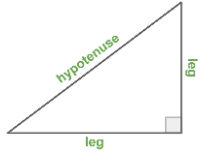# Lengths of Squares and Right Triangles

## Learning Goal

Students will explore relationships between areas and side lengths of squares, right triangles, and composite shapes, and learn how to find the length of the hypotenuse and the distance between two points.For a right triangle, the hypotenuse is the long side, and the legs are the two shorter sides. So far, we know the hypotenuse length is always greater than either of the leg lengths and less than both leg lengths added together.

## Question 1: Use the visual to...

1. For each square, find the side lengths.
2. For each right triangle, find the leg lengths and estimate the hypotenuse length.
Hint: The area of the movable square is known, and for a square , so .

## Question 2: Use the Visual to...

1. Find the area of the fixed square.
2. Find the side lengths of the fixed square.
Hint: Use squares and/or right triangles to compose the fixed square.

## Question 3: Use the Visual to...

Find the hypotenuse length of Triangle 1. Hint: You will do something similar to what you did in Question 2. Notice that the hypotenuse is the side of the inner square.

## Question 4: Use the Visual to...

Find the distance between each set of two points.

1. (2, 2) and (4, 4)
2. (-4, 6) and (1, -4)
Hint 1: The hypotenuse is the side of the square and the distance. Hint 2: Use a composition of shapes to find the area of the square, so you can find the side length.